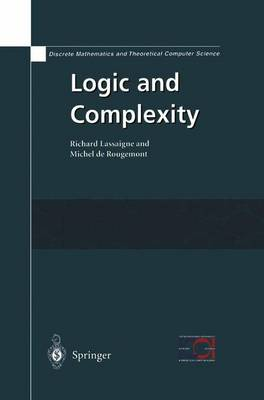•# Logic and Complexity - Discrete Mathematics and Theoretical Computer Science (Hardback)

(author), (author)
£129.99
Hardback 361 Pages
Published: 20/01/2004
Email me when available

Stay one step ahead and let us notify you when this item is next available to order

Logic and Complexity looks at basic logic as it is used in Computer Science, and provides students with a logical approach to Complexity theory. With plenty of exercises, this book presents classical notions of mathematical logic, such as decidability, completeness and incompleteness, as well as new ideas brought by complexity theory such as NP-completeness, randomness and approximations, providing a better understanding for efficient algorithmic solutions to problems.

Divided into three parts, it covers:

- Model Theory and Recursive Functions - introducing the basic model theory of propositional, 1st order, inductive definitions and 2nd order logic. Recursive functions, Turing computability and decidability are also examined.

- Descriptive Complexity - looking at the relationship between definitions of problems, queries, properties of programs and their computational complexity.

- Approximation - explaining how some optimization problems and counting problems can be approximated according to their logical form.

Logic is important in Computer Science, particularly for verification problems and database query languages such as SQL. Students and researchers in this field will find this book of great interest.

Publisher: Springer London Ltd
ISBN: 9781852335656
Number of pages: 361
Weight: 1550 g
Dimensions: 235 x 155 x 22 mm
Edition: 2004 ed.